# 自动选择有 "钱途" 的信号

5 二月 2018, 08:12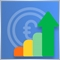0
8 969

### 交易信号的评级模型

1)交易账户的杠杆。

• 20 分 将被赋予杠杆 1:1
• 0 分 w将被赋予杠杆 1:500 及以上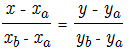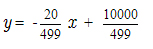2)账户增长百分比。

• 负增长意味着信号目前处于危险之中, 因此将得到 0 分。
• 替代评估绝对增长, 我们通过将账户增长除以信号生存期来衡量其随时间的变化。
• 风险是一个主观的概念。每周增长 10％ 对一些交易者来说可能是不够的, 而对其他人来说这是一个良好的增长率。不过, 我们需要设定一定的范围。因此, 1％ 的增长率将被作为保守信号的参考值, 15％ 将被设定为风险交易的门槛。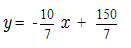3)最大回撤百分比。

• 最高 20％ 的回撤将被视为保守交易风格。它的分数将等于 20。
• 40％ 以上的回撤率将被认为是有风险的, 并被给予 0 分。
• 最大回撤的 20-40％ 的区间将基于之前两个点的直线方程进行评估。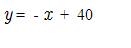4)ROI (投资回报) 百分比

• ROI 低于 100% 则赋予 0 分。
• ROI 高于 200% 则赋予 20 分。
• 100-200 的间隔将根据之前两个点的直线方程进行评估。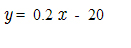5)交易信号生存期。

• 生存期低于 4 周的信号 (一个月内完整周数) 将给予 0 分。
• 生存期 25 周以上 (六个月内的完整周数) 将给予 25 分。
• 4 至 25 周的间隔将根据上述两点设定的直线方程进行评估。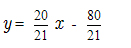### 信号评估工具的实现

• 终端中当前账户的可用信号列表。
• 列表中所选信号按类别详细评估。
• 当前账户可用交易信号的数量。
• 在列表中所选交易信号的可视汇总得分。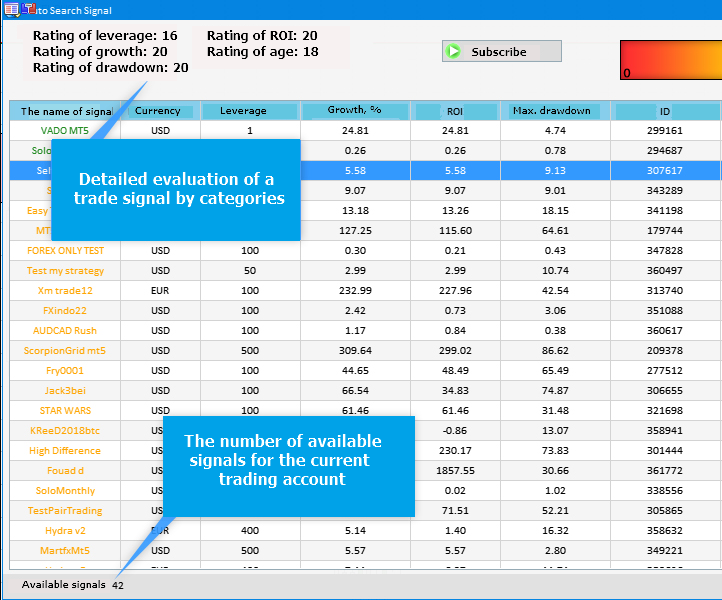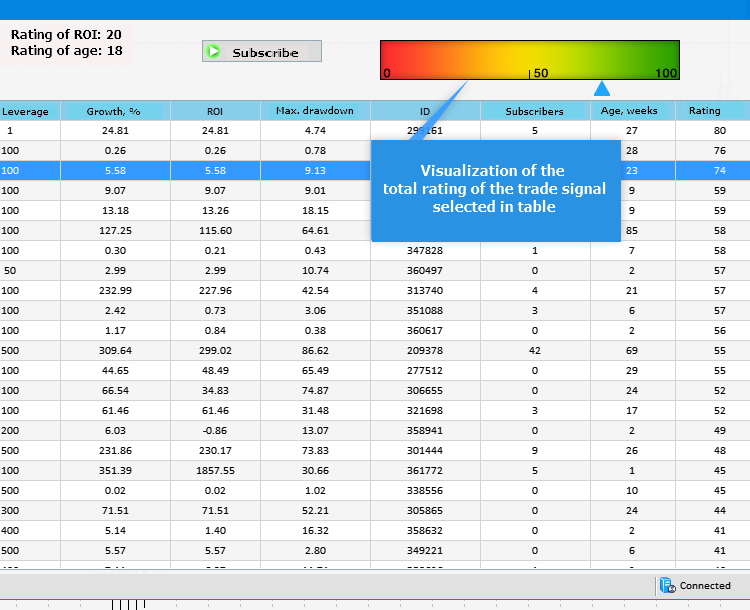• CreateWindow() 方法创建带有标题的应用程序窗口。它可以被关闭和最小化。
• CreateTable() 创建一个包含当前账户所有可用信号的表格。
• CreateStatusBar() 方法创建一个状态栏, 显示可用交易信号的总数。
• CreatePicture1()CreatePicture2() 分别创建渐变比例和比例指针。
• CreateTextLabel() 方法设置显示有关所选交易信号评级的详细信息。
• CreateButton() 方法创建一个按钮, 用于订阅表中选择的信号。
```//+------------------------------------------------------------------+
//| 创建程序的图形界面                                                   |
//+------------------------------------------------------------------+
bool CProgram::CreateGUI(void)
{
//--- 创建面板
if(!CreateWindow("自动搜索信号"))
return(false);
//--- 创建表格
if(!CreateTable(7,100))
return(false);
//--- 状态栏
if(!CreateStatusBar(1,26))
return(false);
//--- 图像
if(!CreatePicture1(618,40))
return(false);
if(!CreatePicture2(610,80))
return(false);
//--- 文本标签
if(!CreateTextLabel1(20,20,"杠杆得分: -"))
return(false);
if(!CreateTextLabel2(20,40,"增长得分: -"))
return(false);
if(!CreateTextLabel3(20,60,"回撤得分: -"))
return(false);
if(!CreateTextLabel4(200,20,"投资回报得分: -"))
return(false);
if(!CreateTextLabel5(200,40,"生存期得分: -"))
return(false);
//--- 图标按钮
if(!CreateButton(440,40,"订阅"))
return(false);
//--- 用户图形界面创建完成
CWndEvents::CompletedGUI();
return(true);
}
//+-----------------------------------------------------------------

```

```//+------------------------------------------------------------------+
//| 事件处理器                                                         |
//+------------------------------------------------------------------+
void CProgram::OnEvent(const int id,const long &lparam,const double &dparam,const string &sparam)
{
//--- 列表或清单项的按击事件
if(id==CHARTEVENT_CUSTOM+ON_CLICK_LIST_ITEM)
{
int x=610+3*int(m_table.GetValue(9,m_table.SelectedItem()));
//---
CreateTextLabel1(20,20,"杠杆得分: "+IntegerToString(GetLeverageRating(int(m_table.GetValue(2,m_table.SelectedItem())))));
CreateTextLabel2(20,40,"增长得分: "+IntegerToString(GetGainRating(int(m_table.GetValue(3,m_table.SelectedItem())),int(m_table.GetValue(8,m_table.SelectedItem())))));
CreateTextLabel3(20,60,"回撤得分: "+IntegerToString(GetDrawDownRating(int(m_table.GetValue(5,m_table.SelectedItem())))));
CreateTextLabel4(200,20,"投资回报得分: "+IntegerToString(GetROIRating(int(m_table.GetValue(4,m_table.SelectedItem())))));
CreateTextLabel5(200,40,"生存期得分: "+IntegerToString(GetWeeksRating(int(m_table.GetValue(8,m_table.SelectedItem())))));
CreatePicture2(x,80);
//---
m_button.IsLocked(false);
Update(true);
}
//---
if(id==CHARTEVENT_CUSTOM+ON_CLICK_BUTTON)
{
//--- 如果按钮被按下
if(lparam==m_button.Id())
{
SignalSubscribe(long(m_table.GetValue(6,m_table.SelectedItem())));
}
}
}

```

```//+------------------------------------------------------------------+
//| 接收有关可用交易信号的信息                                            |
//+------------------------------------------------------------------+
{
//--- 请求信号数据库中的信号总数
m_signals_total=SignalBaseTotal();
//---
ArrayResize(m_name,m_signals_total);
ArrayResize(m_curr,m_signals_total);
ArrayResize(m_leverage,m_signals_total);
ArrayResize(m_gain,m_signals_total);
ArrayResize(m_roi,m_signals_total);
ArrayResize(m_max_drawdown,m_signals_total);
ArrayResize(m_pips,m_signals_total);
ArrayResize(m_subscr,m_signals_total);
ArrayResize(m_weeks,m_signals_total);
ArrayResize(m_rating,m_signals_total);
//--- 遍历所有信号
for(int i=0;i<m_signals_total;i++)
{
//--- 选择一个信号进一步操作
if(SignalBaseSelect(i))
{
//--- 接收信号属性
m_name[i]=SignalBaseGetString(SIGNAL_BASE_NAME);                                                // 信号名称
m_curr[i]=SignalBaseGetString(SIGNAL_BASE_CURRENCY);                                            // 信号的货币
m_leverage[i]=SignalBaseGetInteger(SIGNAL_BASE_LEVERAGE);                                       // 杠杆
m_gain[i]=SignalBaseGetDouble(SIGNAL_BASE_GAIN);                                                // 账户增长 %
m_roi[i]=SignalBaseGetDouble(SIGNAL_BASE_ROI);                                                  // 投资回报
m_max_drawdown[i]=SignalBaseGetDouble(SIGNAL_BASE_MAX_DRAWDOWN);                                // 最大回撤
m_id[i]=SignalBaseGetInteger(SIGNAL_BASE_ID);                                                   // 信号 ID
m_subscr[i]=SignalBaseGetInteger(SIGNAL_BASE_SUBSCRIBERS);                                      // 订阅者数量
//--- 接收总评分
m_rating[i]=GetLeverageRating(m_leverage[i])+GetGainRating(m_gain[i],m_weeks[i])+GetDrawDownRating(m_max_drawdown[i])+GetROIRating(m_roi[i])+GetWeeksRating(m_weeks[i]);
}
else
{
PrintFormat("信号选择错误. 错误代码=%d",GetLastError());
return(false);
}
}
return (true);
}
//+------------------------------------------------------------------+

```

```//+------------------------------------------------------------------+
//| 交易杠杆评估                                                       |
//+------------------------------------------------------------------+
int CProgram::GetLeverageRating(long leverage)
{
int lev_rating=int(-20.0/499.0*double(leverage)+10000.0/499.0);
lev_rating=(lev_rating>20)?20:lev_rating;
return lev_rating;
}
//+------------------------------------------------------------------+
//| 增长率评估                                                         |
//+------------------------------------------------------------------+
int CProgram::GetGainRating(double gain,int weeks)
{
weeks=(weeks==0)?1:weeks;
int gain_rating=int(-10*(gain/double(weeks)/7.0)+150.0/7.0);
gain_rating=(gain_rating>20)?20:gain_rating;
gain_rating=(gain_rating<0)?0:gain_rating;
gain_rating=(gain<0)?0:gain_rating;
return gain_rating;
}
//+------------------------------------------------------------------+
//| 最大回撤评估                                                       |
//+------------------------------------------------------------------+
int CProgram::GetDrawDownRating(double max_drawdown)
{
int drawdn_rating=int(-max_drawdown+40);
drawdn_rating=(drawdn_rating>20)?20:drawdn_rating;
drawdn_rating=(drawdn_rating<0)?0:drawdn_rating;
return drawdn_rating;
}
//+------------------------------------------------------------------+
//| 投资回报评估                                                       |
//+------------------------------------------------------------------+
int CProgram::GetROIRating(double roi)
{
int roi_rating=int(0.2*roi-20);
roi_rating=(roi_rating>20)?20:roi_rating;
roi_rating=(roi_rating<0)?0:roi_rating;
return roi_rating;
}
//+------------------------------------------------------------------+
//| 交易信号生存时间评估                                                 |
//+------------------------------------------------------------------+
int CProgram::GetWeeksRating(int weeks)
{
int age_rating=int(20.0*double(weeks)/21.0-80.0/21.0);
age_rating=(age_rating>20)?20:age_rating;
age_rating=(age_rating<0)?0:age_rating;
return age_rating;
}

```

```//+------------------------------------------------------------------+
//| 初始化表格                                                         |
//+------------------------------------------------------------------+
void CProgram::InitializingTable(void)
{
//---
string columns=
{
"信号名称",
"账户货币",
"账户杠杆",
"账户增长, %",
"投资回报",
"最大回撤",
"信号 ID",
"订阅者数量",
"生存时间, 周",
"评分"
};
//---
for(int c=0; c<COLUMNS1_TOTAL; c++)
{
//--- 设置表头标题
//---
for(int r=0; r<m_signals_total; r++)
{
if(c==0)
m_table.SetValue(c,r,m_name[r]);
else if(c==1)
m_table.SetValue(c,r,m_curr[r]);
else if(c==2)
m_table.SetValue(c,r,IntegerToString(m_leverage[r]));
else if(c==3)
m_table.SetValue(c,r,DoubleToString(m_gain[r],2));
else if(c==4)
m_table.SetValue(c,r,DoubleToString(m_roi[r],2));
else if(c==5)
m_table.SetValue(c,r,DoubleToString(m_max_drawdown[r],2));
else if(c==6)
m_table.SetValue(c,r,IntegerToString(m_id[r]));
else if(c==7)
m_table.SetValue(c,r,IntegerToString(m_subscr[r]));
else if(c==8)
m_table.SetValue(c,r,IntegerToString(m_weeks[r]));
else if(c==9)
m_table.SetValue(c,r,IntegerToString(m_rating[r]));
}
}
}
//+------------------------------------------------------------------+
//| 创建渲染表格                                                       |
//+------------------------------------------------------------------+
#resource "\\Images\\EasyAndFastGUI\\Icons\\bmp16\\arrow_up.bmp"
#resource "\\Images\\EasyAndFastGUI\\Icons\\bmp16\\arrow_down.bmp"
#resource "\\Images\\EasyAndFastGUI\\Icons\\bmp16\\circle_gray.bmp"
#resource "\\Images\\EasyAndFastGUI\\Icons\\bmp16\\calendar.bmp"
//---
bool CProgram::CreateTable(const int x_gap,const int y_gap)
{
#define COLUMNS1_TOTAL 10
//--- 将指针存储到主控件
m_table.MainPointer(m_window);
//--- 列宽数组
int width[COLUMNS1_TOTAL];
::ArrayInitialize(width,110);
width=80;
width=100;
width=90;
width=85;
width=90;
//--- 在列中文本沿 X 轴偏移的数组
int text_x_offset[COLUMNS1_TOTAL];
::ArrayInitialize(text_x_offset,7);
//--- 列中的文本对齐数组
ENUM_ALIGN_MODE align[COLUMNS1_TOTAL];
::ArrayInitialize(align,ALIGN_CENTER);
//---
//--- 属性
m_table.XSize(1000);
m_table.YSize(470);
m_table.CellYSize(20);
m_table.TableSize(COLUMNS1_TOTAL,m_signals_total);
m_table.TextAlign(align);
m_table.ColumnsWidth(width);
m_table.TextXOffset(text_x_offset);
m_table.LabelXGap(5);
m_table.LabelYGap(4);
m_table.IconXGap(7);
m_table.IconYGap(4);
m_table.MinColumnWidth(0);
m_table.IsSortMode(true);
m_table.LightsHover(true);
m_table.SelectableRow(true);
m_table.IsWithoutDeselect(true);
m_table.ColumnResizeMode(true);
m_table.IsZebraFormatRows(clrWhiteSmoke);
m_table.AutoXResizeMode(true);
m_table.AutoXResizeRightOffset(7);
m_table.AutoYResizeBottomOffset(28);
m_table.DataType(2,TYPE_INT);
m_table.DataType(3,TYPE_FLOAT);
m_table.DataType(4,TYPE_FLOAT);
m_table.DataType(5,TYPE_FLOAT);
m_table.DataType(6,TYPE_INT);
m_table.DataType(7,TYPE_INT);
m_table.DataType(8,TYPE_INT);
m_table.DataType(9,TYPE_INT);

//--- 用数据填充表格
InitializingTable();
//--- 创建控件
if(!m_table.CreateTable(x_gap,y_gap))
return(false);
//--- 将该对象添加到对象组的公用数组中
m_table.SortData(9);
m_table.SortData(9);
return(true);
}

```

```//+------------------------------------------------------------------+
//| 创建一个渐变比例                                                    |
//+------------------------------------------------------------------+
#resource "\\Images\\EasyAndFastGUI\\Icons\\bmp64\\000.bmp"
#resource "\\Images\\EasyAndFastGUI\\Controls\\ArrowUp_blue.bmp"
//---
bool CProgram::CreatePicture1(const int x_gap,const int y_gap)
{
//--- 将指针存储到主控件
m_picture1.MainPointer(m_window);
//--- 属性
m_picture1.XSize(300);
m_picture1.YSize(40);
m_picture1.IconFile("Images\\EasyAndFastGUI\\Icons\\bmp64\\000.bmp");
//--- 创建按钮
if(!m_picture1.CreatePicture(x_gap,y_gap))
return(false);
//--- 在基类中添加一个指向元素的指针
return(true);
}
//+------------------------------------------------------------------+
//| 为比例创建一个指针                                                  |
//+------------------------------------------------------------------+
bool CProgram::CreatePicture2(const int x_gap,const int y_gap)
{
//--- 将指针存储到主控件
m_picture2.MainPointer(m_window);
//--- 属性
m_picture2.XSize(16);
m_picture2.YSize(16);
m_picture2.IconFile("Images\\EasyAndFastGUI\\Controls\\ArrowUp_blue.bmp");
//--- 创建按钮
if(!m_picture2.CreatePicture(x_gap,y_gap))
return(false);
//--- 在基类中添加一个指向元素的指针
return(true);
}

```

### 潜在地有 "钱途" 信号的选择

• 第一类别。红色区域。来自该区域的信号可能具有较高的盈利能力, 但涉及高风险。
• 第二类别。黄色区域。这些信号表现出良好的盈利能力, 风险适中。
• 第三类别。绿色区域。这些信号被认为是潜在的有希望的, 因为它们表现出更高的可靠性, 尽管盈利能力可能不高。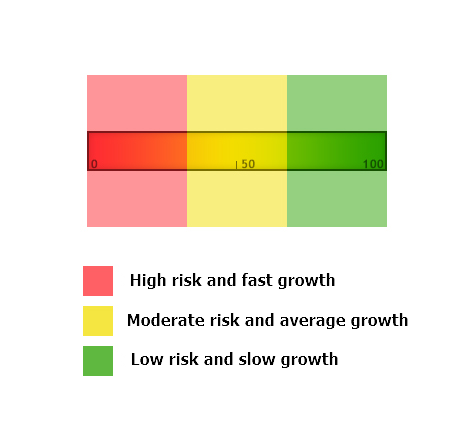```//+------------------------------------------------------------------+
//| 初始化表格                                                         |
//+------------------------------------------------------------------+
void CProgram::InitializingTable(void)
{
//---
string columns=
{
"信号名称",
"账户货币",
"账户杠杆",
"账户增长, %",
"投资回报",
"最大回撤",
"信号 ID",
"订阅者数量",
"生存时间, 周",
"评分"
};
//---
for(int c=0; c<COLUMNS1_TOTAL; c++)
{
//--- 设置表头标题

//---
for(int r=0; r<m_signals_total; r++)
{
if(c==0)
{
m_table.SetValue(c,r,m_name[r]);
if(m_rating[r]<=30)
m_table.TextColor(c,r,clrCrimson);
else if(m_rating[r]>30 && m_rating[r]<=66)
m_table.TextColor(c,r,clrOrange);
else if(m_rating[r]>66)
m_table.TextColor(c,r,clrForestGreen);
}
else if(c==1)
m_table.SetValue(c,r,m_curr[r]);
else if(c==2)
m_table.SetValue(c,r,IntegerToString(m_leverage[r]));
else if(c==3)
m_table.SetValue(c,r,DoubleToString(m_gain[r],2));
else if(c==4)
m_table.SetValue(c,r,DoubleToString(m_roi[r],2));
else if(c==5)
m_table.SetValue(c,r,DoubleToString(m_max_drawdown[r],2));
else if(c==6)
m_table.SetValue(c,r,IntegerToString(m_id[r]));
else if(c==7)
m_table.SetValue(c,r,IntegerToString(m_subscr[r]));
else if(c==8)
m_table.SetValue(c,r,IntegerToString(m_weeks[r]));
else if(c==9)
m_table.SetValue(c,r,IntegerToString(m_rating[r]));
}
}
}

```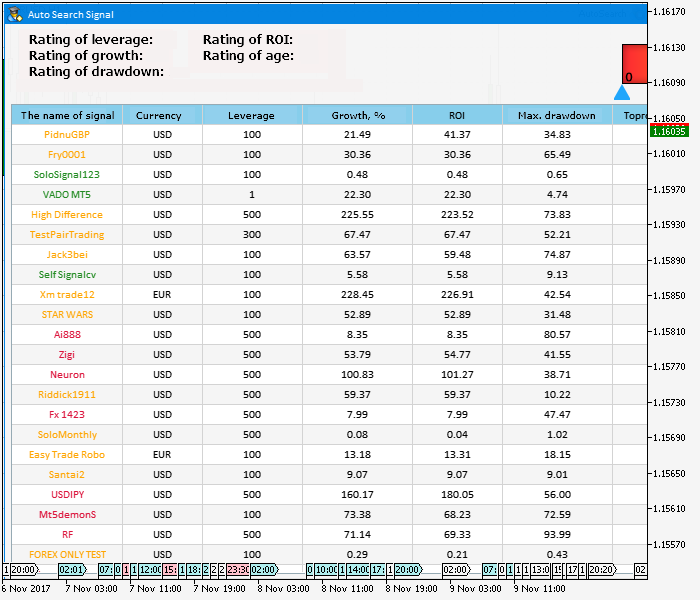```//+------------------------------------------------------------------+
//| 创建一个图像按钮                                                    |
//+------------------------------------------------------------------+
#resource "\\Images\\EasyAndFastGUI\\Icons\\bmp16\\start.bmp"
//---
bool CProgram::CreateButton(const int x_gap,const int y_gap,const string text)
{
//--- 将指针存储到主控件
m_button.MainPointer(m_window);
//--- 属性
m_button.XSize(120);
m_button.YSize(22);
m_button.IconXGap(3);
m_button.IconYGap(3);
m_button.FontSize(10);
m_button.IsCenterText(true);
m_button.IconFile("Images\\EasyAndFastGUI\\Icons\\bmp16\\start.bmp");
m_button.IsLocked(true);
//--- 创建控件
if(!m_button.CreateButton(text,x_gap,y_gap))
return(false);
//--- 在基类中添加一个指向元素的指针
return(true);
}
//+------------------------------------------------------------------+

```

1. 您必须在 MetaTrader 5 中使用您的 mql5 账户登录才能使用终端中的交易信号: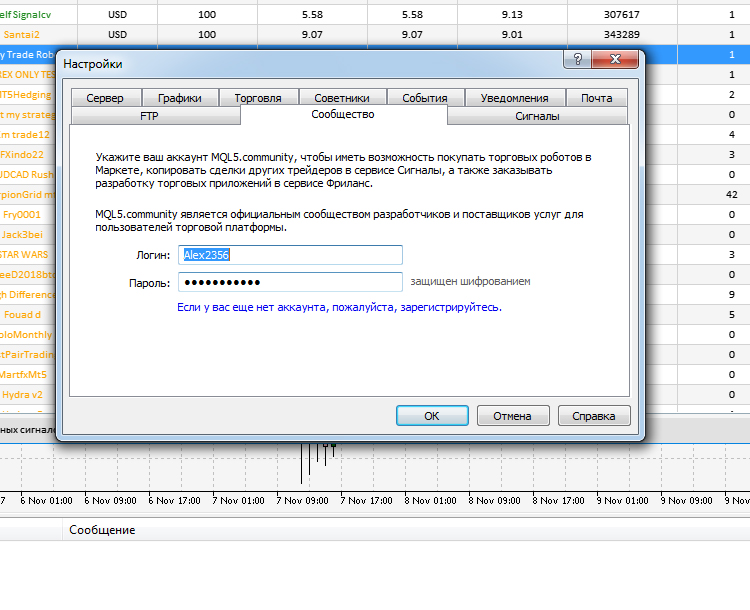2. 当您启动应用程序时, 启用 允许更改信号设置 选项。否则使用此应用程序订阅交易信号将返回错误 4014 ("Function is not allowed for call" - 函数不允许调用)。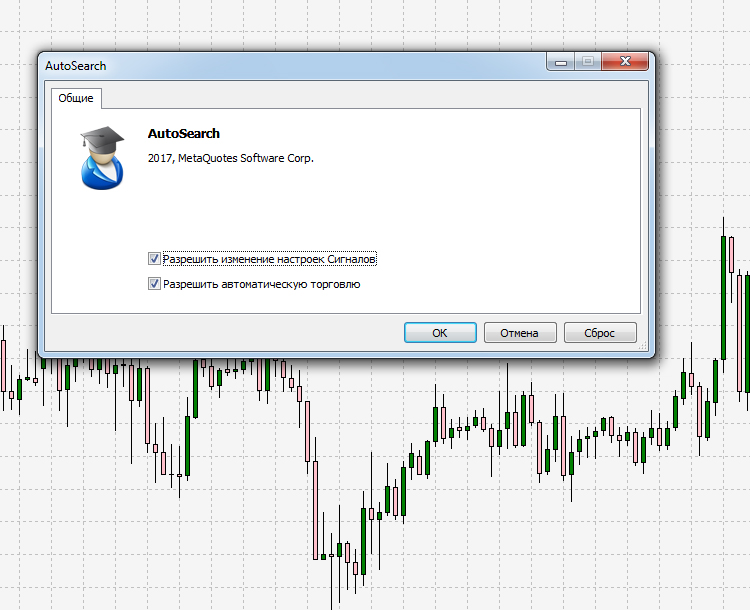3. 最后一个会影响应用程序正常运行的是 signals.dat 文件。如果表格中的数据显示不正确或根本没有显示, 您应该在 C:\Users\用户名\AppData\Roaming\MetaQuotes\Terminal\..\bases\signals 找到并删除它。然后重新启动终端并打开信号选项卡, 如图例 6 所示。一个新的文件将自动生成, 之后您可以启动和使用该应用程序。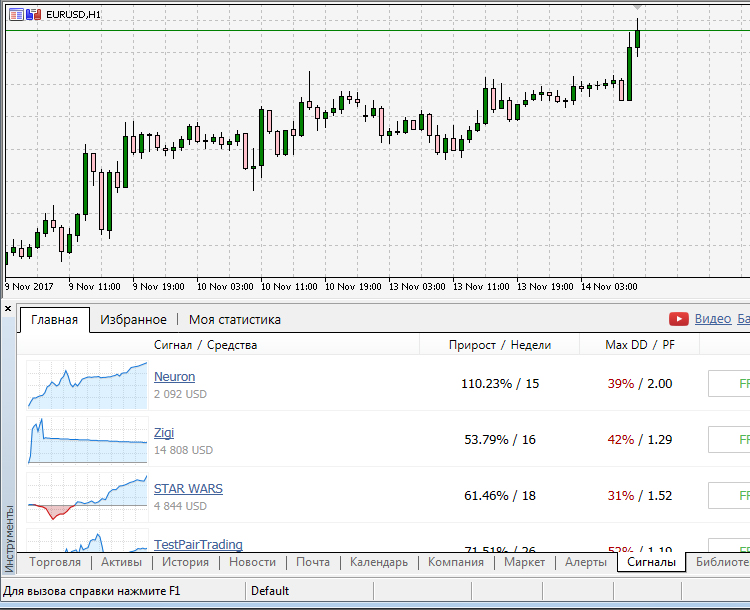### 结束语

#
名称

1
AutoSearch.mq5 智能交易系统
自动选择有 "钱途" 信号的应用程序
2
Program.mqh 代码库  创建应用程序的类
3
MainWindow.mqh 代码库  一组用于创建应用程序的方法

MQL5.zip (1616.79 KB)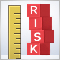单一资产交易顺序中的风险评估. 续篇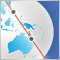在亚洲市场进行夜间交易: 如何保持盈利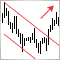通道突破形态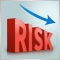如何降低交易者的风险